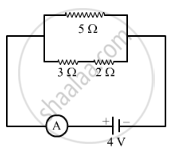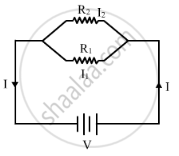Share

# (A) with the Help of a Circuit Diagram, Obtain the Relation for the Equivalent Resistance of Two Resistances Connected in Parallel. (B) in the Circuit Diagram Shown Below, Find: (I) Total - CBSE Class 10 - Science

#### Question

(a) With the help of a circuit diagram, obtain the relation for the equivalent resistance of two resistances connected in parallel.
(b) In the circuit diagram shown below, find:

(i) Total resistance.
(ii) Current shown by the ammeter#### SolutionLet the individual resistance of the two resistors be R1 and R2 and their combined resistance be R. Let the total current flowing in the circuit be I and strength of the battery be volts. Then, from Ohm's law, we have:
V = IR ... (1)
We know that when resistors are connected in parallel, the potential drop across each resistance is the same.
Therefore:
I  = I1 I2
I = V/R1 + V/R2
I = V/(1/R1 + 1/R2) ... (2)
From the equations (1) and (2) we have:
1/R = 1/R1 + 1/R2

As shown Resistance 2Ω and 3Ω are in senies

We know resistance in series arrangement can be obtained as R=R_1+R_2

R=2Ω+3Ω=5Ω

$\text{ Since this 5 \Omega is in parallel with another 5 \Omega resistance therefore total resistance can be obtained as }$

1/R=1/R_1+1/R_2   "Here"  R_1=5Ω R_2=5Ω

1/R=1/5+1/5

$\frac{1}{R} = \frac{2}{5}$
$R = 2 . 5 \Omega$
"Total resistance of combination = 2 . 5 \Omega}"

The current shown by the ammeter A, i.e. the current in the circuit can be calculated as:

I=V/R

I=4/2.5=1.6A

Is there an error in this question or solution?

#### Video TutorialsVIEW ALL 

Solution (A) with the Help of a Circuit Diagram, Obtain the Relation for the Equivalent Resistance of Two Resistances Connected in Parallel. (B) in the Circuit Diagram Shown Below, Find: (I) Total Concept: Electric Current and Circuit.
S